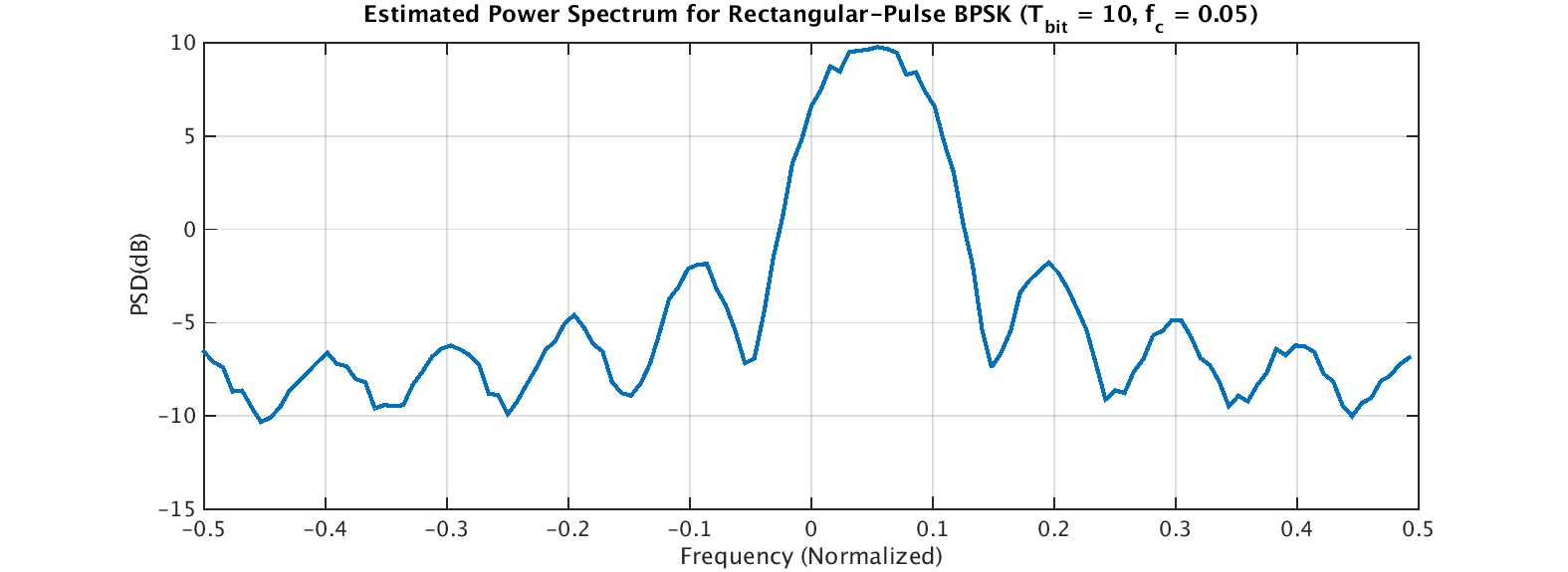# Creating a Simple CS Signal: Rectangular-Pulse BPSK

To test the correctness of various CSP estimators, we need a sampled signal with known cyclostationary parameters. Additionally, the signal should be easy to create and understand. A good candidate for this kind of signal is the binary phase-shift keyed (BPSK) signal with rectangular pulse function.

PSK signals with rectangular pulse functions have infinite bandwidth because the signal bandwidth is determined by the Fourier transform of the pulse, which is a sinc() function for the rectangular pulse. So the rectangular pulse is not terribly practical–infinite bandwidth is bad for other users of the spectrum. However, it is easy to generate, and its statistical properties are known.

So let’s jump in. The baseband BPSK signal is simply a sequence of binary ($\pm$ 1) symbols convolved with the rectangular pulse. The MATLAB script make_rect_bpsk.m does this and produces the following plot:The signal alternates between amplitudes of +1 and -1 randomly. After frequency shifting and adding white Gaussian noise, we obtain the power spectrum estimate:The power spectrum plot shows why the rectangular-pulse BPSK signal is not popular in practice. The range of frequencies for which the signal possesses non-zero average power is infinite, so it will interfere with signals “nearby” in frequency. However, it is a good signal for us to use as a test input in all of our CSP algorithms and estimators.

The MATLAB script that creates the BPSK signal and the plots above is here. It is an m-file but I’ve stored it in a .doc file due to WordPress limitations I can’t yet get around.I'm a signal processing researcher specializing in cyclostationary signal processing (CSP) for communication signals. I hope to use this blog to help others with their cyclo-projects and to learn more about how CSP is being used and extended worldwide.

Posted on

## 2 thoughts on “Creating a Simple CS Signal: Rectangular-Pulse BPSK”

1.Amitoz says:

Hello,
Thanks for creating this blog.
I am supposed to work on applying deep learning methods for denoising or separating cyclostationary signals. My background is mainly in machine learning, image processing and computer vision. Since I am not from Digital Signal Processing or Digital Communication background, It would be great if you can recommend a or few resources to grasp the basics of these first.

1.Chad Spooner says:

Thank you for your interest in the CSP Blog!

I suppose since your background contains image processing, you do actually have a lot of the basics of DSP for communication-signal processing, such as transform theory, filtering/convolution, and perhaps even spectrum estimation. So I’m thinking what you are currently lacking is more in the areas of mathematical modeling of communication signals and the theory and practice of cyclostationary signal processing.

On CSP, check out my papers in My Papers and, perhaps more importantly, the papers on CSP in The Literature. You might also read/skim all the posts here at the CSP Blog, in their order of appearance (because I start out slowly and build up to more advanced topics).

For communication-signal modeling, a good textbook is Proakis (The Literature [R44]). I’m sure there are online things too. Perhaps Khan Academy?

Finally, for full disclosure, see evidence of my current attitude toward the Machine Learners and their foray into CSP: